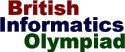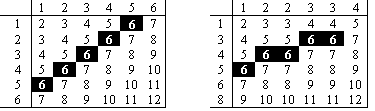The British Informatics Olympiad isthe computing competition for schools and colleges. We are proud to present BIO 2000.The 2000 British Informatics Olympiad - Round One

 Question 3 Playing games note: 'die' is the singular of 'dice' Romulus and Remus are playing a game where they take it in turns to throw a pair of dice and then add the two numbers. In order to make the game fair, the possible scores from Romulus' pair of dice and Remus' dice, and the number of different ways each of them can be formed, must be the same. Every side of a die consists of a single positive integer. [NB: This excludes 0.] For example, Romulus has a normal pair of six sided dice (each numbered {1,2,3,4,5,6}) and Remus has an unusual pair of dice: the first numbered {1,2,2,3,3,4}, and the second numbered {1,3,4,5,6,8}. This is fair because both pairs of dice can make the numbers from 2 to 12, and (for example) 6 can be made in 5 ways with each pair.3 (a) [20 marks] Write a program that inputs the values on one of the pairs of dice and then outputs a different pair of dice that could be used to play a fair game. Two dice are only different if they have different sides; so {1,2,3,4,5,6} and {6,5,4,3,2,1} are not different. Similarly, a different order does not distinguish two pairs of dice; so {1,2,3} and {4,5,6} is not different to {4,5,6} and {1,2,3}. The first line of input will consist of a single integer 'n' (1 <= n <= 8) denoting the number of sides all the dice will have. The second line will consist of 'n' integers (in the range 1 to 8) giving the values on the sides of the first die. The third line will also consist of 'n' integers (in the range 1 to 8) giving the values for the second die. Your output should consist of an example solution if you believe there to be one (with one die given on each line), or the word Impossible if you do not. Note that the example solution does not need to be sorted, and the value on a side can be any positive integer (i.e. values may be greater than 8). Sample run 6 2 4 1 3 5 6 6 4 2 3 1 5   1 2 2 3 3 4 8 6 5 4 3 1
 3 (b) [4 marks] Three equivalent pairs of dice have been mixed together. The six dice are labelled {1,5,6,7,9,10}, {2,3,4,6,7,8}, {2,6,7,8,10,11}, {1,2,3,5,6,7}, {3,7,8,9,11,12} and {3,4,5,7,8,9}. What were the original pairings? 3 (c) [4 marks] Given two 'n' sided dice, what is the largest number of distinct sums that can be formed? What is the smallest?

Total marks: 100.
End of BIO 2000 Round One paper